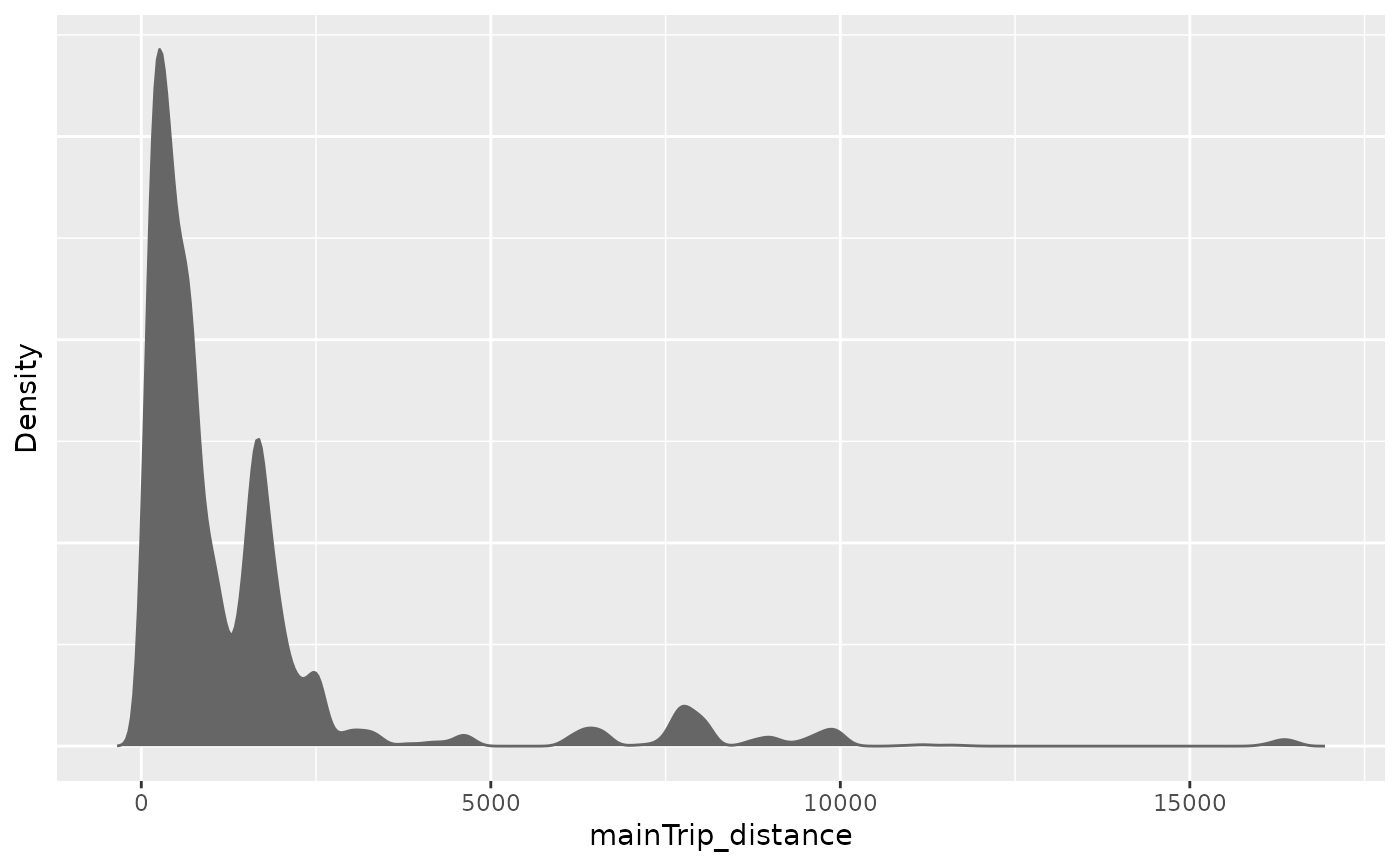Create a density plot or a boxplot of one metric variable or a barplot of one categorical variable, based on a specific subset of the data.

## Usage

plot_density(
dat,
y_var,
plot_type = "density",
apc_range = NULL,
highlight_diagonals = NULL,
y_var_cat_breaks = NULL,
y_var_cat_labels = NULL,
weights_var = NULL,
log_scale = FALSE,
xlab = NULL,
ylab = NULL,
legend_title = NULL,
...
)

## Arguments

dat

Dataset with columns period and age and the main variable specified through argument y_var.

y_var

Character name of the main variable to be plotted.

plot_type

One of c("density","boxplot"). Only used if the y_var column is metric.

apc_range

Optional list with one or multiple elements with names "age","period","cohort" to filter the data. Each element should contain a numeric vector of values for the respective variable that should be kept in the data. All other values are deleted.

highlight_diagonals

Optional internal parameter which is only specified when plot_density is called from within plot_densityMatrix. See plot_densityMatrix for details.

y_var_cat_breaks

Optional numeric vector of breaks to categorize y_var based on calling function cut. Only used to highlight the categories based on different colors. And only used if the y_var column is numeric.

y_var_cat_labels

Optional character vector for the names of the categories that were defined based on y_var_cat_breaks. The length of this vector must be one shorter than length(y_var_cat_breaks). Only used if the y_var column is numeric.

weights_var

Optional character name of a weights variable used to project the results in the sample to some population.

log_scale

Indicator if the main variable should be log10 transformed. Only used if the y_var column is numeric. Defaults to FALSE.

xlab, ylab, legend_title

Optional plot annotations.

...

Additional arguments passed to density.

ggplot object

## Details

If plot_density is called internally from within plot_densityMatrix (i.e., if the dataset contains some of the columns c("age_group","period_group","cohort_group")), this function will calculate the metric densities individually for these groups.

## Author

Alexander Bauer alexander.bauer@stat.uni-muenchen.de, Maximilian Weigert maximilian.weigert@stat.uni-muenchen.de

## Examples

library(APCtools)
data(travel)

plot_density(dat = travel, y_var = "mainTrip_distance")
#> Excluding 9565 missing observations of mainTrip_distance...plot_density(dat = travel, y_var = "mainTrip_distance")
#> Excluding 9565 missing observations of mainTrip_distance...